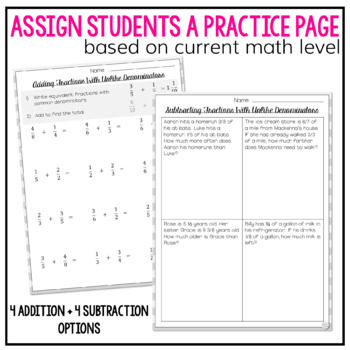# Adding and Subtracting Fractions with Unlike Denominators WorksheetsSubject
Resource Type
File Type

PDF

(904 KB|11 pages)
Standards
Also included in:
1. Adding and subtracting fractions doesn't come easy without a lot of targeted practice. These worksheets are differentiated so you can easily reach your students who need the support of a representative model and so that you can stretch your learners who are ready to apply their understanding of frac
\$6.00
\$4.99
Save \$1.01
2. This bundle includes both printable worksheets and digital activities for use with Google Slides to support your students in adding and subtracting fractions. Print OptionThese worksheets are differentiated so you can easily reach your students who need the support of a representative model and so t
\$10.00
\$7.00
Save \$3.00
3. This bundle includes both printable worksheets and Boom Decks so you will have printable and digital options to support your students in adding and subtracting fractions. Print OptionThese worksheets are differentiated so you can easily reach your students who need the support of a representative mo
\$14.00
\$9.50
Save \$4.50
• Product Description
• StandardsNEW

Students need a ton of practice adding and subtracting fractions with unlike denominators. These worksheets are differentiated so you can easily reach your students who need the support of a representative model and so that you can stretch your learners who are ready to apply their understanding of fractions to word problems.

Included in this resource you will find 8 worksheets including:

-Adding fractions with a visual representation drawn

-Adding fractions with space for students to draw in a visual representation

-Adding fractions with no visual representation

-Subtracting fractions with a visual representation drawn

-Subtracting fractions with space for students to draw in a visual representation

-Subtracting fractions with no visual representation

-Subtracting fractions word problems

Looking for fractions with like AND unlike denominators? These worksheets are a part of a bundle that can be found HERE.

And remember, follow my store so you will be updated when new resources are added! I list many products on sale for the first 24 hours!

Looking for other fraction resources? Check them out HERE!

Solve word problems involving addition and subtraction of fractions referring to the same whole, including cases of unlike denominators, e.g., by using visual fraction models or equations to represent the problem. Use benchmark fractions and number sense of fractions to estimate mentally and assess the reasonableness of answers. For example, recognize an incorrect result 2/5 + 1/2 = 3/7, by observing that 3/7 < 1/2.
Add and subtract fractions with unlike denominators (including mixed numbers) by replacing given fractions with equivalent fractions in such a way as to produce an equivalent sum or difference of fractions with like denominators. For example, 2/3 + 5/4 = 8/12 + 15/12 = 23/12. (In general, 𝘢/𝘣 + 𝘤/𝘥 = (𝘢𝘥 + 𝘣𝘤)/𝘣𝘥.)
Solve word problems involving addition and subtraction of fractions referring to the same whole and having like denominators, e.g., by using visual fraction models and equations to represent the problem.
Add and subtract mixed numbers with like denominators, e.g., by replacing each mixed number with an equivalent fraction, and/or by using properties of operations and the relationship between addition and subtraction.
Understand addition and subtraction of fractions as joining and separating parts referring to the same whole.
Total Pages
11 pages
N/A
Teaching Duration
N/A
Report this Resource to TpT
Reported resources will be reviewed by our team. Report this resource to let us know if this resource violates TpT’s content guidelines.